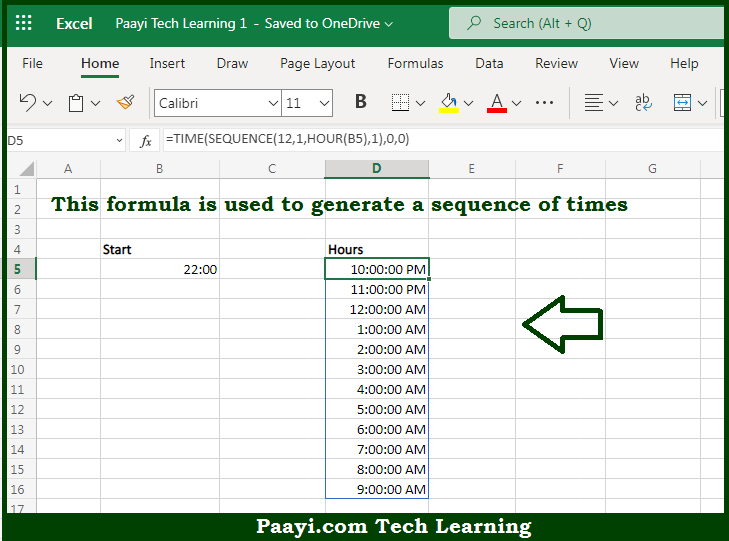# Learn How to Get Sequence of Times in Microsoft Excel

Written by | 0 Comments | 423 Views

In this article, you will learn how to evaluate things in Dynamic Arrays with formulas in Microsoft Excel using a single/combination(s) of functions. You will also know How to Get a Sequence of Times and see the generic formula.

Learn How to Get Sequence of Times in Microsoft Excel

The main purpose of this formula is to generate a sequence of times. Here we will learn how to get the sequence of times in the given data range in the workbook in Microsoft Excel. That implies, with the help of a formula based on the SEQUENCE, TIME, HOUR, MINUTE, and SECOND functions you can able to generate a sequence of times. So, with the help of this formula, you can able to get the sequence of times in the given data range in the workbook in Microsoft Excel.

General Formula to Get Sequence of Times

=TIME(SEQUENCE(n,1,HOUR(start),1),0,0)

The Explanation to Get Sequence of TimesSo we know that with the help of the given formula above you can able to generate a sequence of times. Here we will learn how to get the sequence of times in the given data range in the workbook in Microsoft Excel. As we know that the SEQUENCE function is a dynamic array function that is used to generate multiple results. In the example provided here we are using the TIME function, to create a valid Excel time with hours, minutes, and seconds given as decimal values. So that to create the numbers used for hours with SEQUENCE function. So, with the help of this formula, you can able to generate a sequence of times. So now you have learned how to get the sequence of times in the given data range in the workbook in Microsoft Excel.